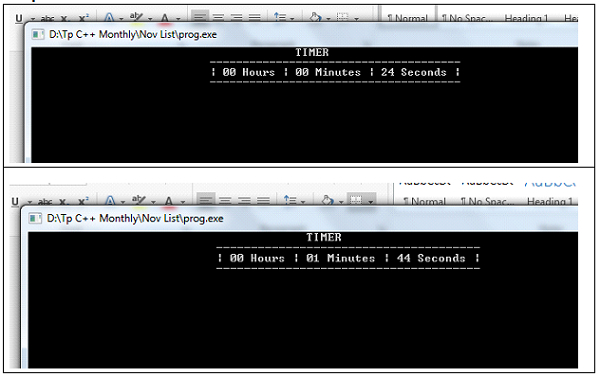# Timer in C++ using system calls

Here we will see how to design timer in C++ using a system call. We will not use any graphics or animations. Here timer means the stopwatch, that is up-counting the time. The used system calls are −

sleep(n) − This will help the program to sleep for n number of seconds

system() − This is used to execute the system command by passing command as an argument to this function.

## Example

Live Demo

#include <iomanip>
#include <iostream>
#include <stdlib.h>
#include <unistd.h>
using namespace std;
int hrs = 0;
int mins = 0;
int sec = 0;
void showClk() {
system("cls");
cout << setfill(' ') << setw(55) << " TIMER \n";
cout << setfill(' ') << setw(66) << " --------------------------------------\n";
cout << setfill(' ') << setw(29);
cout << "| " << setfill('0') << setw(2) << hrs << " Hours | ";
cout << setfill('0') << setw(2) << mins << " Minutes | ";
cout << setfill('0') << setw(2) << sec << " Seconds |" << endl;
cout << setfill(' ') << setw(66) << " --------------------------------------\n";
}
void systemCallTimer() {
while (true) {
showClk();
sleep(1);
sec++;
if (sec == 60) {
mins++;
if (mins == 60) {
hrs++;
mins = 0;
}
sec = 0;
}
}
}
int main() {
systemCallTimer();
}

## Output